Home Practice
For learners and parents For teachers and schools
Textbooks
Full catalogue
Pricing SupportLog in

We think you are located in United States. Is this correct?

# 2.2 Force

## 2.2 Force (ESBKH)

### What is a force?(ESBKJ)

A force is anything that can cause a change to objects. Forces can do things like:

• change the shape of an object,

• accelerate or stop an object, and

• change the direction of a moving object.

A force can be classified as either a contact force or a non-contact force.

A contact force must touch or be in contact with an object to cause a change. Examples of contact forces are:

• the force that is used to push or pull things, like on a door to open or close it

• the force that a sculptor uses to turn clay into a pot

• the force of the wind to turn a windmill

A non-contact force does not have to touch an object to cause a change. Examples of non-contact forces are the forces due to:

• gravity, like the Earth pulling the Moon towards itself;

• electricity, like a proton and an electron attracting each other; and

• magnetism, like a magnet pulling a paper clip towards itself.

The unit of force in the international system of units (S.I. units) is the newton (symbol N). This unit is named after Sir Isaac Newton who first defined force. Force is a vector quantity and so it has a magnitude and a direction. We use the symbol $$\overrightarrow{F}$$ for force.

This chapter will often refer to the resultant force acting on an object. The resultant force is simply the vector sum of all the forces acting on the object. It is very important to remember that all the forces must be acting on the same object. The resultant force is the force that has the same effect as all the other forces added together.

### Different types of forces in physics (ESBKK)

A lot of the physics topics you will study revolve around the impact or effect of forces. Although there are many different forces, will learn some fundamental principles for approaching the problems and applications in this book no matter which force applies

Physics is the study of the natural world and you probably know a lot more physics than you think. You see things happening everyday that are governed by the laws of physics but you probably aren't thinking about physics at the time. If you throw a stone up in the air it eventually falls to the ground. A lot of physics can be learnt by analysing an everyday situation.

We are going to learn about some forces in the next few sections but before we start lets describe an everyday situation in which they all play a role so you can visualise what is happening. You need a table and a three books that all have different masses. Take any book and put it on the table. Nothing happens, the book just rests on the table if the table is flat. If you slowly lift one side of the table so that the top of the table is tilted the book doesn't move immediately. As you lift the table more and more the book suddenly starts to slide off the table. You can repeat this with all three books and see how much you have to tilt the table before the books start to slide.

This real world situation illustrates a lot of the physics we want to learn about in this chapter.

#### The normal force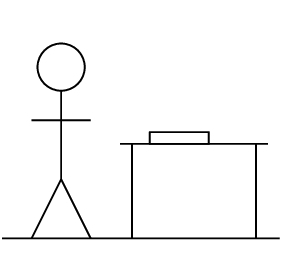When an object is placed on a surface, for example think of the case of putting a book on a table, there are a number of forces acting. Firstly, if the table were not there the book would fall to the floor. The force that causes this is gravity. The table stops the book falling to the floor. The only way this can happen is for the table to exert a force on the book. The force that the table exerts on the book must balance out the force of gravity. This tells us a few things immediately! Gravity is a force pulling the book down, it is a vector. The force that the table exerts must balance this out and it can only do this if it has the same magnitude and acts in the opposite direction.

This occurs often, gravity pulls a person towards the earth but when you are standing on the ground something must be balancing it, if you put a heavy box on the ground the gravitational force is balanced. If you put a brick on water it will sink because nothing balances the gravitational force. We give the force that a surface (any surface) exerts to balance the forces on an object in contact with that surface the normal force.

The normal force is a force that acts on the object as a result of the interaction with the surface and is perpendicular to the surface. This last part might be seem unexpected (counter-intuitive) because if we tilt the table slightly the direction of the gravitational force hasn't changed but the direction of the normal force has a little (the normal is not always directly opposite gravity). Don't panic, this will all make sense before the end of this chapter. Remember: the normal force is always perpendicular (at a right angle) to the surface.

Normal force
The normal force, $$\vec{N}$$, is the force exerted by a surface on an object in contact with it.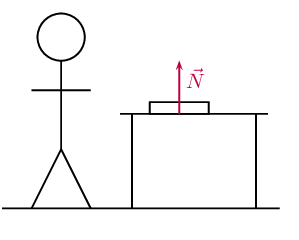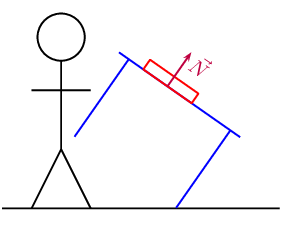temp text

#### Friction forces

Why does a box sliding on a surface eventually come to a stop? The answer is friction. Friction arises where two surfaces are in contact and moving relative to each other.

For an everyday example, press your hands together and move one backwards and forwards, we have two surfaces in contact with one moving relative to the other. Your hands get warm, you will have experienced this before and probably rub your hands together in winter to warm them up. The heat is generated through friction.

Friction arises because the surfaces interact with each other. Think about sandpaper with lots of bumps on the surface. If you rub sandpaper the bumps will slot into any groove .

When the surface of one object slides over the surface of another, each body exerts a frictional force on the other. For example if a book slides across a table, the table exerts a frictional force onto the book and the book exerts a frictional force onto the table. Frictional forces act parallel to surfaces.

Frictional force
Frictional force is the force that opposes the motion of an object in contact with a surface and it acts parallel to the surface the object is in contact with.

The magnitude of the frictional force depends on the surface and the magnitude of the normal force. Different surfaces will give rise to different frictional forces, even if the normal force is the same. Frictional forces are proportional to the magnitude of the normal force. $F_{\text{friction}} \propto N$

For every surface we can determine a constant factor, the coefficient of friction, that allows us to calculate what the frictional force would be if we know the magnitude of the normal force. We know that static friction and kinetic friction have different magnitudes so we have different coefficients for the two types of friction:

• $$\mu_s$$ is the coefficient of static friction
• $$\mu_k$$ is the coefficient of kinetic friction

A force is not always large enough to make an object move, for example a small applied force might not be able to move a heavy crate. The frictional force opposing the motion of the crate is equal to the applied force but acting in the opposite direction. This frictional force is called static friction. When we increase the applied force (push harder), the frictional force will also increase until it reaches a maximum value. When the applied force is larger than the maximum force of static friction the object will move. The static frictional force can vary from zero (when no other forces are present and the object is stationary) to a maximum that depends on the surfaces.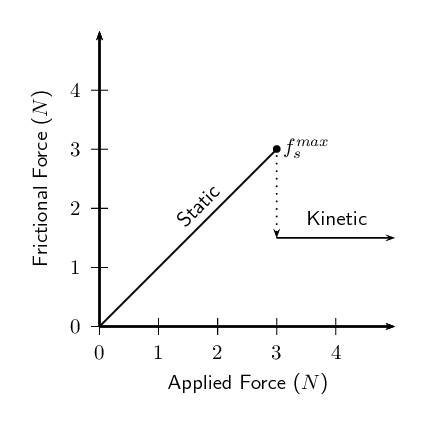For static friction the force can vary up to some maximum value after which friction has been overcome and the object starts to move. So we define a maximum value for the static friction: $$f_s^{max} = \mu_sN$$.

When the applied force is greater than the maximum, static frictional force, the object moves but still experiences friction. This is called kinetic friction. For kinetic friction the value remains the same regardless of the magnitude of the applied force. The magnitude of the kinetic friction is: $$f_k = \mu_kN$$.

Remember that static friction is present when the object is not moving and kinetic friction while the object is moving. For example when you drive at constant velocity in a car on a tar road you have to keep the accelerator pushed in slightly to overcome the friction between the tar road and the wheels of the car. However, while moving at a constant velocity the wheels of the car are rolling, so this is not a case of two surfaces “rubbing” against each other and we are in fact looking at static friction. If you should break hard, causing the car to skid to a halt, we would be dealing with two surfaces rubbing against each other and hence kinetic friction. The higher the value for the coefficient of friction, the more 'sticky' the surface is and the lower the value, the more 'slippery' the surface is.

Friction is very useful. If there was no friction and you tried to prop a ladder up against a wall, it would simply slide to the ground. Rock climbers use friction to maintain their grip on cliffs. The brakes of cars would be useless if it wasn't for friction!

## Worked example 1: Static friction

A box resting on a surface experiences a normal force of magnitude $$\text{30}$$ $$\text{N}$$ and the coefficient of static friction between the surface and the box, $$\mu_s$$, is $$\text{0,34}$$. What is the maximum static frictional force?

### Maximum static friction

We know that the relationship between the maximum static friction, $$f_s^{max}$$, the coefficient of static friction, $$\mu_s$$ and the normal, $$N$$, to be:$f_s^{max} = \mu_sN$

We have been given that $$\mu_s = \text{0,34}$$ and $$N=\text{30}\text{ N}$$. This is all of the information required to do the calculation.

### Calculate the result

\begin{align*} f_s^{max} &= \mu_sN \\ &= (\text{0,34})(\text{30}) \\ & = \text{10,2} \end{align*}

The maximum magnitude of static friction is $$\text{10,2}$$ $$\text{N}$$.

## Worked example 2: Static friction

The forwards of your school's rugby team are trying to push their scrum machine. The normal force exerted on the scrum machine is $$\text{10 000}$$ $$\text{N}$$. The machine isn't moving at all. If the coefficient of static friction is $$\text{0,78}$$ what is the minimum force they need to exert to get the scrum machine to start moving?

### Minimum or maximum

The question asks what the minimum force required to get the scrum machine moving will be. We don't know a relationship for this but we do know how to calculate the maximum force of static friction. The forwards need to exert a force greater than this so the minimum amount they can exert is in fact equal to the maximum force of static friction.

### Maximum static friction

We know that the relationship between the maximum static friction, $$f_s^{max}$$, the coefficient of static friction, $$\mu_s$$ and the normal, $$N$$, to be:$f_s^{max} = \mu_sN$

We have been given that $$\mu_s = \text{0,78}$$ and $$N=\text{10 000}\text{ N}$$. This is all of the information required to do the calculation.

### Calculate the result

\begin{align*} f_s^{max} &= \mu_sN \\ &= (\text{0,78})(\text{10 000}) \\ & = \text{7 800}\text{ N} \end{align*}

The maximum magnitude of static friction is $$\text{7 800}$$ $$\text{N}$$.

## Worked example 3: Kinetic friction

The normal force exerted on a pram is $$\text{100}$$ $$\text{N}$$. The pram's brakes are locked so that the wheels cannot turn. The owner tries to push the pram but it doesn't move. The owner pushes harder and harder until it suddenly starts to move when the applied force is three quarters of the normal force. After that the owner is able to keep it moving with a force that is half of the force at which it started moving. What is the magnitude of the applied force at which it starts moving and what are the coefficients of static and kinetic friction?

### Maximum static friction

The owner of the pram increases the force he is applying until suddenly the pram starts to move. This will be equal to the maximum static friction which we know is given by: $f_s^{max} = \mu_sN$

We are given that the magnitude of the applied force is three quarters of the normal force magnitude, so: \begin{align*} f_s^{max} &= \frac{\text{3}}{\text{4}}N \\ &= \frac{\text{3}}{\text{4}}(\text{100}) \\ &=\text{75}\text{ N} \end{align*}

### Coefficient of static friction

We now know both the maximum magnitude of static friction and the magnitude of the normal force so we can find the coefficient of static friction: \begin{align*} f_s^{max} &= \mu_sN \\ \text{75} &= \mu_s (\text{100}) \\ \mu_s &=\text{0,75} \end{align*}

### Coefficient of kinetic friction

The magnitude of the force required to keep the pram moving is half of the magnitude of the force required to get it to start moving so we can determine it from: \begin{align*} f_k &= \frac{\text{1}}{\text{2}}f_s^{max} \\ &= \frac{\text{1}}{\text{2}}(\text{75}) \\ & = \text{37,5}\text{ N} \end{align*}

We know the relationship between the magnitude of the kinetic friction, magnitude of the normal force and coefficient of kinetic friction. We can use it to solve for the coefficient of kinetic friction: \begin{align*} f_k &= \mu_kN \\ \text{37,5} &= \mu_k (\text{100}) \\ \mu_k &=\text{0,375} \end{align*}

## Worked example 4: Coefficient of static friction

A block of wood experiences a normal force of $$\text{32}$$ $$\text{N}$$ from a rough, flat surface. There is a rope tied to the block. The rope is pulled parallel to the surface and the tension (force) in the rope can be increased to $$\text{8}$$ $$\text{N}$$ before the block starts to slide. Determine the coefficient of static friction.

### Analyse the question and determine what is asked

The normal force is given ($$\text{32}$$ $$\text{N}$$) and we know that the block does not move until the applied force is $$\text{8}$$ $$\text{N}$$.

We are asked to find the coefficient for static friction $${\mu }_{s}$$.

### Find the coefficient of static friction

\begin{align*} F_f & = \mu_sN \\ \text{8} &= \mu_s(\text{32}) \\ \mu_s &= \text{0,25} \end{align*}

Note that the coefficient of friction does not have a unit as it shows a ratio. The value for the coefficient of friction friction can have any value up to a maximum of $$\text{0,25}$$. When a force less than $$\text{8}$$ $$\text{N}$$ is applied, the coefficient of friction will be less than $$\text{0,25}$$.

## Worked example 5: Static friction

A box resting on an inclined plane experiences a normal force of magnitude $$\text{130}$$ $$\text{N}$$ and the coefficient of static friction, $$\mu_s$$, between the box and the surface is $$\text{0,47}$$. What is the maximum static frictional force?

### Maximum static friction

We know that the relationship between the maximum static friction, $$f_s^{max}$$, the coefficient of static friction, $$\mu_s$$ and the normal, $$N$$, to be:$f_s^{max} = \mu_sN.$ This does not depend on whether the surface is inclined or not. Changing the inclination of the surface will affect the magnitude of the normal force but the method of determining the frictional force remains the same.

We have been given that $$\mu_s = \text{0,47}$$ and $$N=\text{130}\text{ N}$$. This is all of the information required to do the calculation.

### Calculate the result

\begin{align*} f_s^{max} &= \mu_sN \\ &= (\text{0,47})(\text{130}) \\ & = \text{61,1}\text{ N} \end{align*}

The maximum magnitude of static friction is $$\text{61,1}$$ $$\text{N}$$.

A recommended practical for informal assessment on normal forces and friction is included. In this experiment learners investigate the relationship between the normal force and the maximum static friction. Learners should use different surfaces to see the effect of static friction. If time allows learners can also investigate dynamic friction. You will need spring balances; several blocks, of the same material, with hooks attached to one end; several rough and smooth surfaces; and bricks or blocks to incline the surfaces.

## Normal forces and friction

### Aim

To investigate the relationship between normal force and friction.

### Apparatus

Spring balance, several blocks, of the same material, with hooks attached to one end, several rough and smooth surfaces, bricks or blocks to incline the surfaces

### Method

• Attach each of the blocks to the spring balance in turn and note the reading.

• Take one block and attach it to the spring balance. Now slide the block along each of the surfaces in turn. Note the readings. Repeat for the other blocks.

• Repeat the above step but incline the surfaces at different angles.

### Results

Record your results in a table

Early humans made use of friction to create fire. Friction can create a lot of heat and the early humans used this fact when they rubbed two sticks together to start a fire. When you rub your hands together fast and pressing hard you will feel that they get warm. This is heat created by the friction. You can use this to start a fire.

To start a fire you need two pieces of wood, one long straight, round piece approximately the same thickness as your finger and about $$\text{40}$$ $$\text{cm}$$ long as well as a thicker flat piece of wood. The flat thick piece of wood needs a hole that the long straight one can fit into. Then you put the flat piece on the ground, the long straight one in the hole and rub it between your hands applying downwards pressure to increase the normal force and the amount of friction. Where the two pieces of wood rub against each other the friction results in many tiny pieces of wood being rubbed off and getting hot.

After a while the hole will start to smoke. At this point the smoking wood bits, called the ember, need to be gently tipped out of the hole into a small bed of dry grass. You cover the ember completely and blow gently. The grass should start to burn. Then you use the burning grass to light some dry twigs, and keep working your way up to bigger pieces of wood.

To make it even easier, a bow from wood with a string can be used to cause the wood to turn. By twisting the string from the bow around the long piece of wood it can be driven without requiring a person use their hands.

#### Tension

Tension is the magnitude of the force that exists in objects like ropes, chains and struts that are providing support. For example, there are tension forces in the ropes supporting a child's swing hanging from a tree.

Textbook Exercise 2.1

A box is placed on a rough surface. It has a normal force of magnitude $$\text{120}$$ $$\text{N}$$. A force of $$\text{20}$$ $$\text{N}$$ applied to the right cannot move the box. Calculate the magnitude and direction of the friction forces.

The normal force is given ($$\text{120}$$ $$\text{N}$$) and we know that the block does not move when an applied force of $$\text{20}$$ $$\text{N}$$ is used.

We are asked to find the magnitude and direction of the friction forces.

Since the box is stationary and the applied force is insufficient to move the box, we know that the kinetic friction force is $$\text{0}$$ $$\text{N}$$.

The frictional force is defined as the the force that opposes the motion of an object in contact with a surface and it acts parallel to the surface the object is in contact with. Therefore the static frictional force is $$\text{20}$$ $$\text{N}$$ to the left to give a resultant of zero.

A block rests on a horizontal surface. The normal force is $$\text{20}$$ $$\text{N}$$. The coefficient of static friction between the block and the surface is $$\text{0,40}$$ and the coefficient of dynamic friction is $$\text{0,20}$$.

What is the magnitude of the frictional force exerted on the block while the block is at rest?

The block is not moving and so there is no kinetic frictional force acting on the block. There is also no force applied to the block and so the static frictional force is also zero.

What will the magnitude of the frictional force be if a horizontal force of magnitude $$\text{5}$$ $$\text{N}$$ is exerted on the block?

The block is not moving and so there is no kinetic frictional force acting on the block.

The frictional force is defined as the the force that opposes the motion of an object in contact with a surface and it acts parallel to the surface the object is in contact with. Therefore the static frictional force is $$\text{5}$$ $$\text{N}$$ in the opposite direction to the applied force.

What is the minimum force required to start the block moving?

To find the minimum force required to start the block moving we need to calculate the maximum static friction force:

\begin{align*} F_{s}^{\text{max}} & = \mu_{s} N \\ & = (\text{0,40})(\text{20}) \\ & = \text{8}\text{ N} \end{align*}

So we must apply a force of magnitude greater than $$\text{8}$$ $$\text{N}$$ to start the box moving.

What is the minimum force required to keep the block in motion once it has been started?

To find the minimum force required to keep the block in motion we need to calculate the magnitude of the force of kinetic friction:

\begin{align*} F_{k} & = \mu_{k} N \\ & = (\text{0,20})(\text{20}) \\ & = \text{4}\text{ N} \end{align*}

So we must apply a force of $$\text{4}$$ $$\text{N}$$ to keep the box in motion.

If the horizontal force is $$\text{10}$$ $$\text{N}$$, determine the frictional force.

This force is greater than the force required to start the block moving. So the static frictional force is $$\text{0}$$ $$\text{N}$$.

For kinetic friction the value of the frictional force remains the same regardless of the magnitude of the applied force. So the kinetic frictional force is $$\text{4}$$ $$\text{N}$$.

A lady injured her back when she slipped and fell in a supermarket. She holds the owner of the supermarket accountable for her medical expenses. The owner claims that the floor covering was not wet and meets the accepted standards. He therefore cannot accept responsibility. The matter eventually ends up in court. Before passing judgement, the judge approaches you, a science student, to determine whether the coefficient of static friction of the floor is a minimum of $$\text{0,5}$$ as required. He provides you with a tile from the floor, as well as one of the shoes the lady was wearing on the day of the incident.

Write down an expression for the coefficient of static friction.

$F_{s} \leq \mu_{s}N$

Plan an investigation that you will perform to assist the judge in his judgement. Follow the steps outlined below to ensure that your plan meets the requirements.

1. Formulate an investigation question.

2. Apparatus: List all the other apparatus, except the tile and the shoe, that you will need.

3. A stepwise method: How will you perform the investigation? Include a relevant, labelled diagram.

4. Results: What will you record?

5. Conclusion: How will you interpret the results to draw a conclusion?

The following provides a guideline to what learners should do. This experiment is very similar to the experiment on normal forces and friction given above.

Investigation question: What is the co-efficient of static friction for the tiles in the supermarket for different shoes and weights of people?

Apparatus: Spring balance, several blocks, of the same material, with hooks attached to one end, several rough and smooth surfaces, bricks or blocks to incline the surfaces (and the tile and shoe)

Method:

1. Measure the frictional force for a minimum mass
2. Measure the frictional force for a maximum mass
3. Measure the frictional force for an average mass
4. Repeat each of the above measurements and take the average
5. Determine the average co-efficient of friction

Results: You should record the results for each step described above

Conclusion: If the co-efficient of static friction is less than the minimum required than the supermarket owner is incorrect. If the co-efficient of static friction is greater than the minimum required than the lady is incorrect. If the co-efficient of static friction is the same as the minimum then other factors must be considered.

### Force diagrams (ESBKM)

Force diagrams are sketches of the physical situation you are dealing with, with arrows for all the forces acting drawn on the system. For example, if a block rests on a surface then there is a force from gravity pulling the block down and there is a normal force acting on the block from the surface. The normal force and the force of gravity have the same magnitude in this situation. The force diagram for this situation is: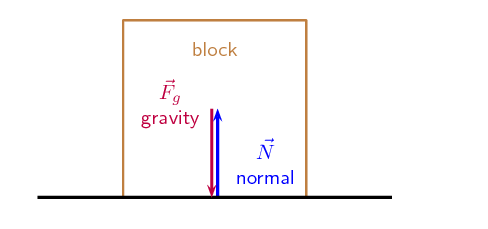The length of the arrows are the same to indicate that the forces have the same magnitude.

Another example could be a the same block on a surface but with an applied force, $$\vec{F}_L$$, to the left of $$\text{10}$$ $$\text{N}$$ and an applied force, $$\vec{F}_R$$, to the right of $$\text{20}$$ $$\text{N}$$. The weight and normal also have magnitudes of $$\text{10}$$ $$\text{N}$$.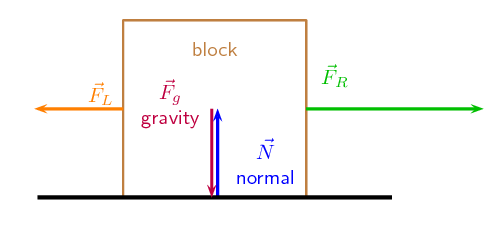It is important to keep the following in mind when you draw force diagrams:

• Make your drawing large and clear.

• You must use arrows and the direction of the arrow will show the direction of the force.

• The length of the arrow will indicate the size of the force, in other words, the longer arrows in the diagram ($${F}_{R}$$ for example) indicates a bigger force than a shorter arrow ($${F}_{L}$$). Arrows of the same length indicate forces of equal size ($${F}_{N}$$ and $${F}_{g}$$). Use “little lines” like in maths to show this.

• Draw neat lines using a ruler. The arrows must touch the system or object.

• All arrows must have labels. Use letters with a key on the side if you do not have enough space on your drawing.

• The labels must indicate what is applying the force (the force of the car) on what the force is applied (on the trailer) and in which direction (to the right)

• If the values of the forces are known, these values can be added to the diagram or key.

### Free body diagrams (ESBKN)

In a free-body diagram, the object of interest is drawn as a dot and all the forces acting on it are drawn as arrows pointing away from the dot. We can redraw the two force diagrams above as free body diagrams: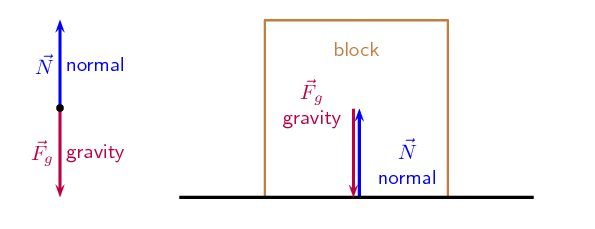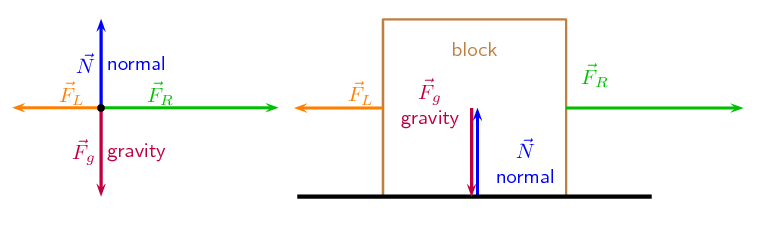temp text

### Resolving forces into components (ESBKP)

We have looked at resolving forces into components. There is one situation we will consider where this is particularly useful, problems involving an inclined plane. It is important because the normal force depends on the component of the gravitational force that is perpendicular to the slope.

Let us consider a block on an inclined plane. The plane is inclined at an angle $$\theta$$ to the horizontal. It feels a gravitational force, $$\vec{F}_g$$, directly downwards. This force can be broken into components that are perpendicular to the plane and parallel to it. This is shown here: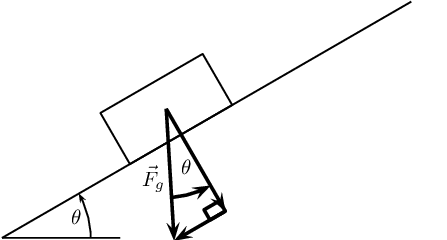We can use any coordinate system to describe the situation and the simplest thing to do is to make the $$x$$-axis of the Cartesian coordinate system align with the inclined plane. Here is the same physical situation with the coordinate system drawn in: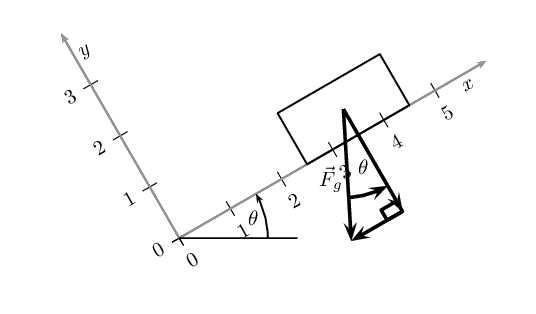This means that the components of gravitational force are aligned (parallel) with one of the axes of the coordinate system. We have shown in the figures that the angle between the horizontal and the incline is also the angle between the gravitational force and its component perpendicular to the inclined plane (this is normal to the plane). Using this angle and the fact that the components form part of a right-angled triangle we can calculate the components using trigonometry: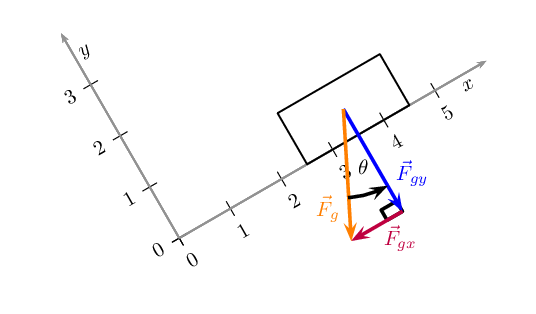Using trigonometry the components are given by: \begin{align*} {F}_{gx} & = {F}_g\sin(\theta)\\ {F}_{gy} & = {F}_g\cos(\theta) \end{align*}

## Worked example 6: Components of force due to gravity

A block on an inclined plane experiences a force due to gravity, $$\vec{F}_g$$ of $$\text{137}$$ $$\text{N}$$ straight down. If the slope is inclined at $$\text{37}$$$$\text{°}$$ to the horizontal, what is the component of the force due to gravity perpendicular and parallel to the slope?

### Components

We know that for a block on a slope we can resolve the force due to gravity, $$\vec{F}_g$$ into components parallel and perpendicular to the slope. \begin{align*} {F}_{gx} & = {F}_g\sin(\theta)\\ {F}_{gy} & = {F}_g\cos(\theta) \end{align*}

### Calculations

This problem is straightforward as we know that the slope is inclined at an angle of $$\text{37}$$$$\text{°}$$. This is the same angle we need to use to calculate the components, therefore: \begin{align*} {F}_{gx} & = {F}_g\sin(\theta)\\ & = (\text{137})\sin(\text{37}\text{°})\\ & = \text{82,45}\text{ N} \end{align*} \begin{align*} {F}_{gy} & = {F}_g\cos(\theta)\\ & = (\text{137})\cos(\text{37}\text{°})\\ & = \text{109,41}\text{ N} \end{align*}

The component of $$\vec{F}_g$$ that is perpendicular to the slope is $$\vec{F}_{gy}$$=$$\text{109,41}$$ $$\text{N}$$ in the negative $$y$$-direction.

The component of $$\vec{F}_g$$ that is perpendicular to the slope is $$\vec{F}_{gx}$$=$$\text{82,45}$$ $$\text{N}$$ in the negative $$x$$-direction.

Textbook Exercise 2.2

A block on an inclined plane experiences a force due to gravity, $$\vec{F}_g$$ of $$\text{456}$$ $$\text{N}$$ straight down. If the slope is inclined at $$\text{67,8}$$$$\text{°}$$ to the horizontal, what is the component of the force due to gravity perpendicular and parallel to the slope?

We know that for a block on a slope we can resolve the force due to gravity, $$\vec{F}_g$$ into components parallel and perpendicular to the slope. \begin{align*} {F}_{gx} & = {F}_g\sin(\theta)\\ {F}_{gy} & = {F}_g\cos(\theta) \end{align*}

The slope is inclined at an angle of $$\text{67,8}$$$$\text{°}$$. This is the same angle we need to use to calculate the components, therefore:

\begin{align*} {F}_{gx} & = {F}_g\sin(\theta)\\ & = (\text{456})\sin(\text{67,8}\text{°})\\ & = \text{422,20}\text{ N} \end{align*} \begin{align*} {F}_{gy} & = {F}_g\cos(\theta)\\ & = (\text{456})\cos(\text{67,8}\text{°})\\ & = \text{172,30}\text{ N} \end{align*}

The component of $$\vec{F}_g$$ that is perpendicular to the slope is $$\vec{F}_{gy}$$=$$\text{172,30}$$ $$\text{N}$$ in the negative $$y$$-direction.

The component of $$\vec{F}_g$$ that is perpendicular to the slope is $$\vec{F}_{gx}$$=$$\text{422,20}$$ $$\text{N}$$ in the negative $$x$$-direction.

A block on an inclined plane is subjected to a force due to gravity, $$\vec{F}_g$$ of $$\text{456}$$ $$\text{N}$$ straight down. If the component of the gravitational force parallel to the slope is $$\vec{F}_{gx}$$=$$\text{308,7}$$ $$\text{N}$$ in the negative $$x$$-direction (down the slope), what is the incline of the slope?

We know that for a block on a slope we can resolve the force due to gravity, $$\vec{F}_g$$ into components parallel and perpendicular to the slope. \begin{align*} {F}_{gx} & = {F}_g\sin(\theta)\\ {F}_{gy} & = {F}_g\cos(\theta) \end{align*}

We are given the horizontal component of gravity and the force due to gravity. We can use these to find the incline of the slope:

\begin{align*} {F}_{gx} & = {F}_g\sin(\theta)\\ \text{308,7} & = (\text{456})\sin \theta\\ \sin \theta & = \text{0,6769} \ldots \\ \theta & = \text{42,61}\text{°} \end{align*}

The slope is at an incline of $$\text{42,61}$$$$\text{°}$$.

### Finding the resultant force (ESBKQ)

The easiest way to determine a resultant force is to draw a free body diagram. Remember from Chapter 1 that we use the length of the arrow to indicate the vector's magnitude and the direction of the arrow to show which direction it acts in.

After we have done this, we have a diagram of vectors and we simply find the sum of the vectors to get the resultant force.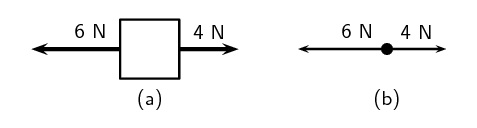Figure 2.4: (a) Force diagram of 2 forces acting on a box. (b) Free body diagram of the box.

For example, two people push on a box from opposite sides with forces of $$\text{4}$$ $$\text{N}$$ and $$\text{6}$$ $$\text{N}$$ respectively as shown in Figure 2.4 (a). The free body diagram in Figure 2.4 (b) shows the object represented by a dot and the two forces are represented by arrows with their tails on the dot.

As you can see, the arrows point in opposite directions and have different lengths. The resultant force is $$\text{2}$$ $$\text{N}$$ to the left. This result can be obtained algebraically too, since the two forces act along the same line. First, as in motion in one direction, choose a frame of reference. Secondly, add the two vectors taking their directions into account.

For the example, assume that the positive direction is to the right, then:\begin{align*} F_R & = (\text{4}) + (-\text{6}) \\ & = -\text{2} \\ & = -\text{2}\text{ N}\ \text{to the left.} \end{align*}

Remember that a negative answer means that the force acts in the opposite direction to the one that you chose to be positive. You can choose the positive direction to be any way you want, but once you have chosen it you must use it consistently for that problem.

As you work with more force diagrams in which the forces exactly balance, you may notice that you get a zero answer (e.g. $$\text{0}$$ $$\text{N}$$). This simply means that the forces are balanced and the resultant is zero.

Once a force diagram has been drawn the techniques of vector addition introduced in Chapter 1 can be used. Depending on the situation you might choose to use a graphical technique such as the tail-to-head method or the parallelogram method, or else an algebraic approach to determine the resultant. Since force is a vector quantity all of these methods apply.

A good strategy is:

• resolve all forces into components parallel to the $$x$$- and $$y$$-directions;
• calculate the resultant in each direction, $$\vec{R}_x$$ and $$\vec{R}_y$$, using co-linear vectors; and
• use $$\vec{R}_x$$ and $$\vec{R}_y$$ to calculate the resultant, $$\vec{R}$$.

## Worked example 7: Finding the resultant force

A car (experiencing a gravitational force of magnitude $$\text{12 000}$$ $$\text{N}$$ and a normal force of the same magnitude) applies a force of $$\text{2 000}$$ $$\text{N}$$ on a trailer (experiencing a gravitational force of magnitude $$\text{2 500}$$ $$\text{N}$$ and normal force of the same magnitude). A constant frictional force of magnitude $$\text{200}$$ $$\text{N}$$ is acting on the trailer, and a constant frictional force of magnitude $$\text{300}$$ $$\text{N}$$ is acting on the car.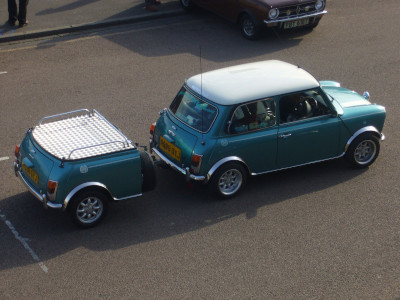1. Draw a force diagram of all the forces acting on the car.

2. Draw a free body diagram of all the forces acting on the trailer.

3. Use the force diagram to determine the resultant force on the trailer.

### Draw the force diagram for the car.

The question asks us to draw all the forces on the car. This means that we must include horizontal and vertical forces.

### Determine the resultant force on the trailer.

To find the resultant force we need to add all the horizontal forces together. We do not add vertical forces as the movement of the car and trailer will be in a horizontal direction, and not up or down. $${F}_{R}=\text{2 000}+\left(-\text{200}\right)=\text{1 800} \text{N to the right}$$.

## Forces and motion

Textbook Exercise 2.3

A boy pushes a shopping trolley (weight due to gravity of $$\text{150}$$ $$\text{N}$$) with a constant force of $$\text{75}$$ $$\text{N}$$. A constant frictional force of $$\text{20}$$ $$\text{N}$$ is present.

Draw a labelled force diagram to identify all the forces acting on the shopping trolley.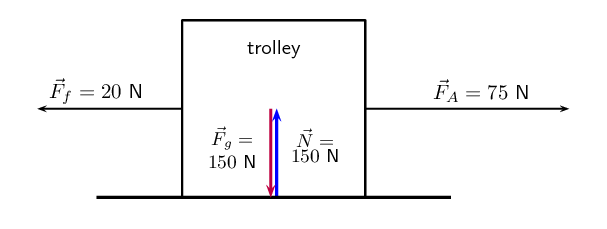Where:

\begin{align*} \vec{F}_{f} & \text{ frictional force} \\ \vec{F}_{A} & \text{ applied force} \\ \vec{F}_{g} & \text{ gravitational force} \\ \vec{N} & \text{ normal force} \end{align*}

Draw a free body diagram of all the forces acting on the trolley.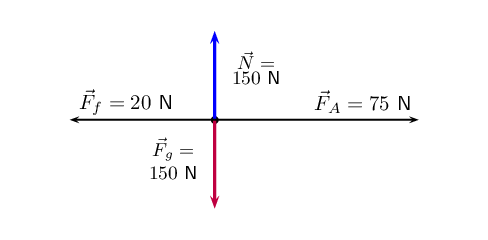Where:

\begin{align*} \vec{F}_{f} & \text{ frictional force} \\ \vec{F}_{A} & \text{ applied force} \\ \vec{F}_{g} & \text{ gravitational force} \\ \vec{N} & \text{ normal force} \end{align*}

Determine the resultant force on the trolley.

The normal force and the gravitational force are balanced and produced no net force on the trolley.

We need to determine the resultant force using the force of friction and the applied force. We choose to the right as positive and assume that the applied force is applied to the right, while the frictional force acts to the left.

\begin{align*} \vec{F}_{R} & = \vec{F}_{f} + \vec{F}_{A} \\ & = -\text{20} + \text{75} \\ & = \text{55}\text{ N} \text{ to the right} \end{align*}

A donkey (experiencing a gravitational force of $$\text{2 500}$$ $$\text{N}$$) is trying to pull a cart (force due to gravity of $$\text{800}$$ $$\text{N}$$) with a force of $$\text{400}$$ $$\text{N}$$. The rope between the donkey and the cart makes an angle of $$\text{30}$$$$\text{°}$$ with the cart. The cart does not move.

Draw a free body diagram of all the forces acting on the donkey.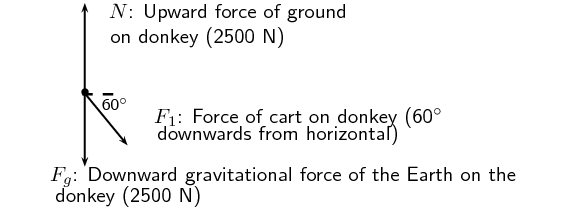Draw a force diagram of all the forces acting on the cart.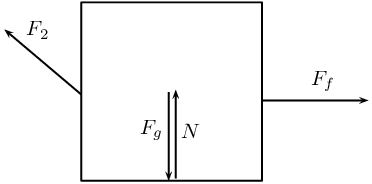Where:

\begin{align*} \vec{F}_{f} & \text{ frictional force} \\ \vec{F}_{2} & \text{ force of cart on donkey} \\ \vec{F}_{g} & \text{ gravitational force} \\ \vec{N} & \text{ normal force} \end{align*}

Find the magnitude and direction of the frictional force preventing the cart from moving.

The cart is not moving so this is static friction only. We note that the frictional force acts in the $$x$$-direction only and will be equal to the $$x$$ component of the force applied by the donkey. The direction will be in the opposite direction that the donkey is pulling. The frictional force is:

\begin{align*} \vec{F}_{f} & = F_{A}\cos \theta \\ & = \text{400} \cos(\text{30}) \\ & = \text{346,41}\text{ N} \text{ in the opposite direction to the applied force} \end{align*}